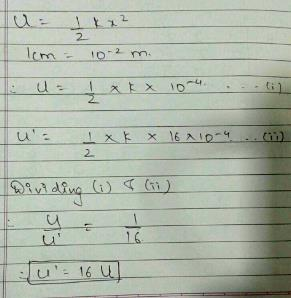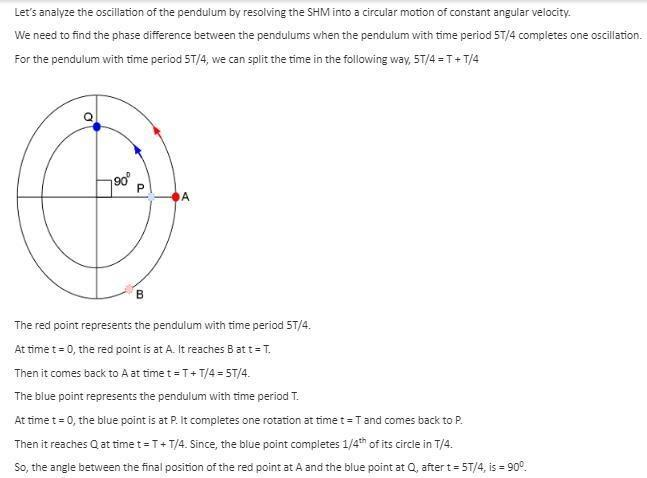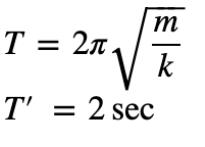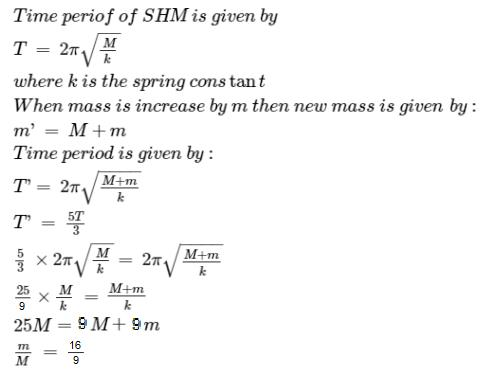Courses

# Test: Oscillations

## 30 Questions MCQ Test Physics Class 11 | Test: Oscillations

Description
This mock test of Test: Oscillations for JEE helps you for every JEE entrance exam. This contains 30 Multiple Choice Questions for JEE Test: Oscillations (mcq) to study with solutions a complete question bank. The solved questions answers in this Test: Oscillations quiz give you a good mix of easy questions and tough questions. JEE students definitely take this Test: Oscillations exercise for a better result in the exam. You can find other Test: Oscillations extra questions, long questions & short questions for JEE on EduRev as well by searching above.
QUESTION: 1

### The pendulum of a wall clock executes

Solution:

The bob of a pendulum moves in such a way that it repeats its positions several time but alternately the time gap between these positions is always equal which proves that the motion of a pendulum is oscillatory.

QUESTION: 2

### In a simple pendulum the restoring force is due to

Solution:

In a simple pendulum the restoring force is due to the tangential component of the gravitational force because on applying torque equation on the radial forces the effect is nullified due to it passing from centre & only tangential component remains.

QUESTION: 3

### The kinetic energy of a body executing S.H.M. is 1/3 of the potential energy. Then, the displacement of the body is x percent of the amplitude, where x is

Solution:

We know that PE + KE = TE = constant
Hence at the extremis TE = PE = ½ kz2
Where z is amplitude and k is shm constant.
Thus when KE = ⅓ PE
We get PE = ¾ TE =  ½ kz2
Hence we get  ½ kx2 = ¾  ½ kz2
We get x/z = √3/4
= 1.73 / 2
= .87
Thus we get x is 87 percent of the amplitude.

QUESTION: 4

If the length of a simple pendulum is increases by 1percent, then the new time-period

Solution:

Time period, T=2π√l/g
=>T∝√l
=>ΔT/T=1/2 Δl/l
Δl/l=1%
Therefore, ΔT/T=1/2x1%=0.5%

QUESTION: 5

A body is executing S.H.M When its displacement from the mean position is 4 cm and 5 cm, the corresponding velocities of the body are 10 cm per sec and 8 cm per sec. Then the time period of the body is

Solution:

v=ω√A2−x2
​⇒8=ω √A2−25​
&10=ω√A2−16
​⇒(8/10​)2= A2−25/ A2−16
​⇒16/25​= A2−25/ A2−16
​⇒16A2−256=25A2−625
⇒9A2=369
A2=41
⇒8=ω41−25
​⇒8=4ω
∴ω=2=T2π​
T=π sec​

QUESTION: 6

Oscillatory motion is

Solution:

Oscillatory motion is periodic to and fro motion. By definition

QUESTION: 7

In a damped system

Solution:
QUESTION: 8

A spring with spring constant k when stretched through 1 cm has a potential energy U. If it is stretched by 4 cm, the potential energy will become

Solution:QUESTION: 9

Two pendulums have time periods T and 5T/4. They are in phase at their mean positions at some instant of time. What will be their phase difference when the bigger pendulum completes one oscillation?

Solution:QUESTION: 10

The ratio of maximum acceleration to maximum velocity of a particle performing S.H.M is equal to

Solution:

Maximum acceleration = w2A
Maximum velocity = wA
Ratio = w2A/wA = w
w = angular velocity
A = amplitude

QUESTION: 11

Periodic motion is

Solution:
QUESTION: 12

Damping is due to

Solution:

Damping is due to resistive forces like air drag, friction etc.

QUESTION: 13

Two pendulums of length 1 m and 16 m start vibrating one behind the other from the same stand. At some instant, the two are in the mean position in the same phase. The time period of shorter pendulum is T. The minimum time after which the two threads of the pendulum will be one behind the other is

Solution:

T1=2π√1/g=T, T2=2π√16/g=4T
(ω1−ω2)t=2π
(2π/T−2π/4T)t=2π
t=4T/3

QUESTION: 14

The period of a simple pendulum is doubled when

Solution:
QUESTION: 15

A particle is executing S.H.M with an amplitude of 4 cm and time period 12 sec. The time taken by the particle in going from its mean position to a position of displacement equal to 2 cm is T1..The time taken from this displaced position of 2 cm to reach the extreme position is T2. Therefore, T1/ T2 will be

Solution:
QUESTION: 16

Period of a periodic motion is the ________ interval of time after which the motion is __________.

Solution:

Explanation:Time period of an oscillatory motion is the smallest interval of time for one complete oscillation.As when we apply the force on the bob,bob moves from mean position to extreme position then form extreme position to mean position and then again from mean to extreme in opposite direction and at last come to mean position.The time taken by bob in this whole process is called period of oscillation.

QUESTION: 17

In simple models damping force is

Solution:
QUESTION: 18

For all practical purposes, the motion of a simple pendulum is SHM,

Solution:

For all practical purposes, the motion of a simple pendulum is SHM only if the maximum angle which the string makes with the vertical is less than 1 because for angles less than 1° sinθ = θ.

QUESTION: 19

Whose motion is an ideal case of SHM?

Solution:

Simple pendulum is not a case of ideal SHM as it is mention in NCERT.
Shadow of a point mass having uniform circular motion on the horizontal diameter is a case of SHM but not on the vertical diameter.

QUESTION: 20

If the period of oscillation of mass M suspended from a spring is one second, then the period of mass 4 M will be

Solution:

T=2*3.14*√m/√k=1 s (given)

T’=2*3.14*√4m/√k=2*3.14*2√m/√k=2*1s

Therefore T’=2sQUESTION: 21

Frequency of the periodic motion is

Solution:
QUESTION: 22

Damped natural frequency is

Solution:

If a resonant mechanical structure is set in motion and left to its own devices, it will continue to oscillate at a particular frequency known as its natural frequency, or "damped natural frequency". This will be a little lower in frequency than the resonant frequency, which is the frequency it would assume if there were no damping. The resonant frequency is also called the "undamped natural frequency”

QUESTION: 23

A rubber ball with water, having a small hole in its bottom is used as the bob of a simple pendulum. The time-period of such a pendulum:

Solution:

When rubber ball completely filled with water its centre of gravity will be at its centre, as water will fall through hole its COG will shift towards lower side leading to increase in length of pendulum and thus T, when very small amount of water will be left in rubber ball its COG will again shifts upward causing decrease in length and thus T, and finally when rubber ball becomes empty its COG will be at its centre and T will remains same as earlier.

QUESTION: 24

A particle executes S.H.M. having time period T, then the time period with which the potential energy changes is:

Solution:
QUESTION: 25

The length of the second’s pendulum on the surface of earth is 1m. The length of second’s pendulum on the surface of moon, where g is 1/6th the value on earth is

Solution:

Time period remains same since
T = 2π √(L/g)
So, from proportionality we can solve

QUESTION: 26

Any periodic function can be expressed as a.

Solution:

All periodic functions can, in principle, be represented as a sum of sine and cosine functions, since they form what's known as a complete set.

QUESTION: 27

In forced oscillations apart from acceleration forces, damping and spring forces there is

Solution:
QUESTION: 28

A mass M is suspended from a spring of negligible mass. The spring is pulled a little and then released so that the mass executes simple harmonic oscillations with a time period T. If the mass is increased by m, the time period becomes 5T/3. then the ratio of m/M is

Solution:QUESTION: 29

The length of a second’s pendulum decreases by 0.1percent, then the clock

Solution:

L’=1.001L
T=2π√L/9=1s
T’=2π√L’/g=2π√1.001/g=Tx√1.001=1.0005xT
T’=1.0005s
Error in time per day=1.0005x60x60x24-1x60x60x24
Error=86443.2-86400
Error=43.2s

QUESTION: 30

If the maximum velocity and acceleration of a particle executing S.H.M are equal in magnitude, the time period will be

Solution: